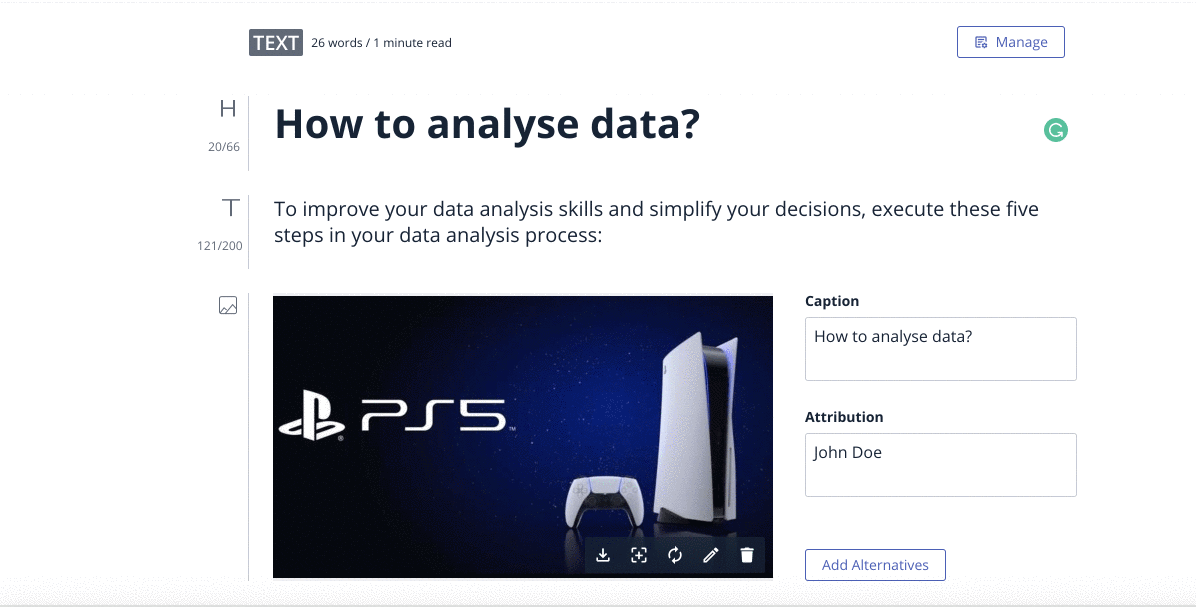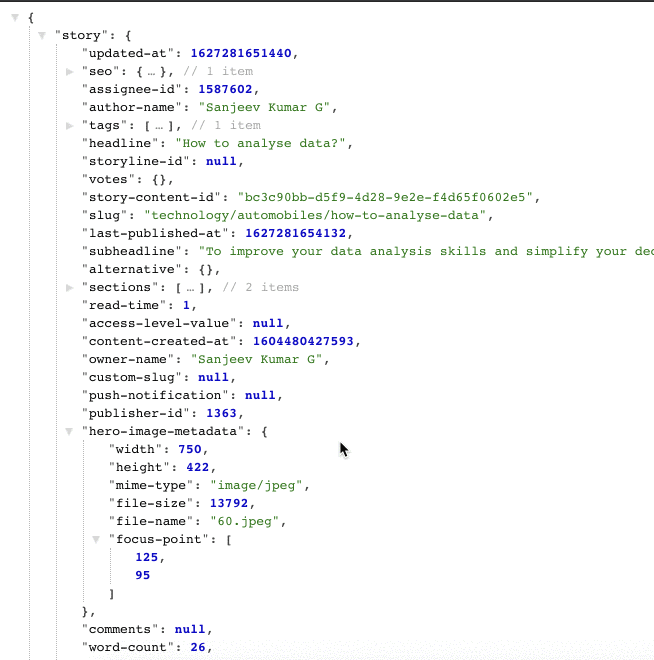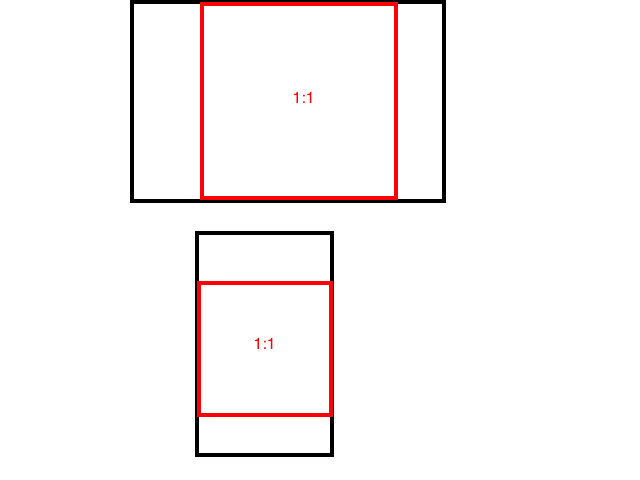Implementing Focus Point using Gumlet

This tutorial was contributed by Athira

Focus points are particularly useful for responsive layouts and to make sure the most important part of the image is always visible regardless of the element size.You can set a focal point to ensure that a key part of an image won’t get cropped. It will ensure that embarrassing image crops do not happen.

How to set image Focus Point in BoldAfter you upload an image, you can click anywhere on that image to define a Focus Point for that image. Focus Point ensures that when an image gets cropped to different sizes, the set Focus Point will always be in the viewport.To remove the Focus Point applied to an image, click the Clear Focus Point button. By default, there is no focus point to be applied.Once you set the Focus Point, in the story API we can see the image metadata which contains the focus-point in an array format.

How to implement Focus Point using Gumlet

Gumlet automatically converts images to the best format and size for the user browser and device.

Extract (rect)

This extract parameter will extract a region from an image. The rect is an alias for this parameter. This operation is always applied before the resize operation. Please give the parameters according to the original image.

The value should be provided as a comma-separated list left,top,width,height.

• left is zero-indexed offset from left edge
• top is zero-indexed offset from top edge
• width of extracted image
• height of extracted image

Example : extract=0,0,300,300, extract=300,300,200,200

How to find the rect value using image Focus Point and Aspect Ratio

We need to find the image bounds (x,y) using the Image Dimensions, Aspect Ratio and Focus Point.

Let’s see how we can find the rect value. First, we need to find out whether we need to take the entire with or height of the image according to the aspect ratio.For that, we need to check the ratio between the image dimensions and the aspect ratio.

Case 1 :

Suppose if ((image width * aspect ratio height) < (image height * aspect ratio width)) is true, we need to find out the expected crop height.

expected crop height = (image width * aspect ratio height)/aspect ratio width

In this case, our rect value will be [0, y, image width, expected crop height ]

Now we need to find the value of y. So that we need to find the image top bound value. For that, we need to check a couple of conditions.

1. if focus point height - (expected crop height/2) < 0 true top bound will be zero

2. if focus point height + (expected crop height/2) > image height true, top bound will be image height - expected crop height/2

3. else top bound will be focus point height - expected crop height/2

After finding the image top bound value, we can find the y value accordingly.

1. Check if top bound + expected crop height > image height true y = image height - expected crop height

2. else y = top bound

Case 2 :

Lets see if (image width * aspect ratio height) < (image height * aspect ratio width) is false, we need to find the expected crop width.

expected crop width = (image height * aspect ratio width )/aspect ratio height

In this case, our rect value will be [x, 0, expected crop width, image height ]

In the next step, we will find the x value, for that we need to find the image left bound value.

1. if focus point width - (expected crop width/2) < 0 true left bound will be zero

2. if focus point width + (expected crop width/2) > image width true, left bound will be image width - expected crop width/2

3. else left bound will be focus point width - expected crop width/2

After finding the image left bound value, we can find the x value accordingly

1. Check if left bound + expected crop width > image width true y = image width - expected crop width

2. else y = left bound

Example:

function imageBounds(imageDimensions =[ 750, 422 ], aspectRatio=[ 16, 9 ], focusPoint=[ 125, 95 ]]) {

// imageDimensions - Image Width and Height
// aspectRatio -  Image Aspect Ratio
// focusPoint - Image Focus Point

var expectedHeight, expectedWidth, bound;

if (imageDimensions * aspectRatio < imageDimensions * aspectRatio) {
// Use the entire width
expectedHeight = (imageDimensions * aspectRatio) / aspectRatio;  //
bound          = findBounds(imageDimensions, expectedHeight, focusPoint);
return [0, Math.round(bound), imageDimensions, Math.round(expectedHeight)];
} else {
// Use the entire height
expectedWidth = (imageDimensions * aspectRatio) / aspectRatio;
bound         = findBounds(imageDimensions, expectedWidth, focusPoint);
return [Math.round(bound), 0, Math.round(expectedWidth), imageDimensions];
}
}
...

function findBounds(imageWidth, cropWidth, focusPoint) {
var leftBound = findLeftBound(imageWidth, cropWidth / 2, focusPoint); 0
if (leftBound + cropWidth > imageWidth) {
return (imageWidth - cropWidth);
}
else {
return leftBound;
}
}
...

function findLeftBound(imageWidth, halfCropWidth, focusPoint) {
if (focusPoint - halfCropWidth < 0) {
return 0;
}
else if (focusPoint + halfCropWidth > imageWidth) {
return imageWidth - halfCropWidth;
}
else {
return focusPoint - halfCropWidth;
}
}

From the above example, we will get the rect value based on the aspect ratio and focus point. Next, we need to pass this rect value as a parameter.

Example: https://demo.gumlet.io/fall.jpeg?rect=0,0,750,422&format=auto